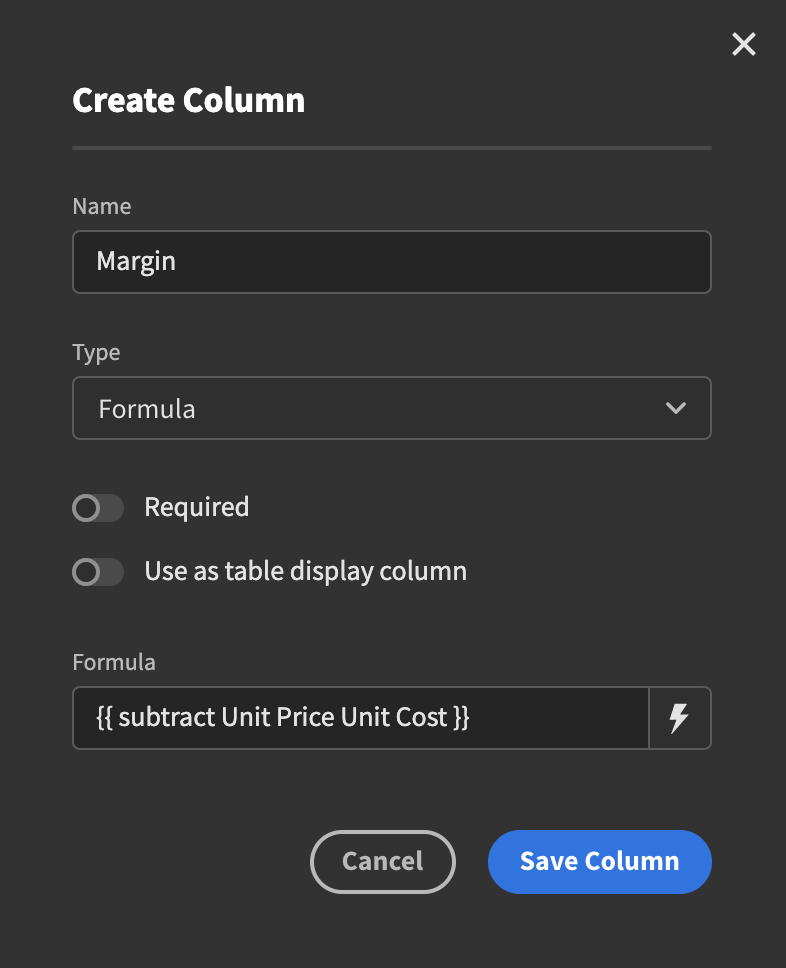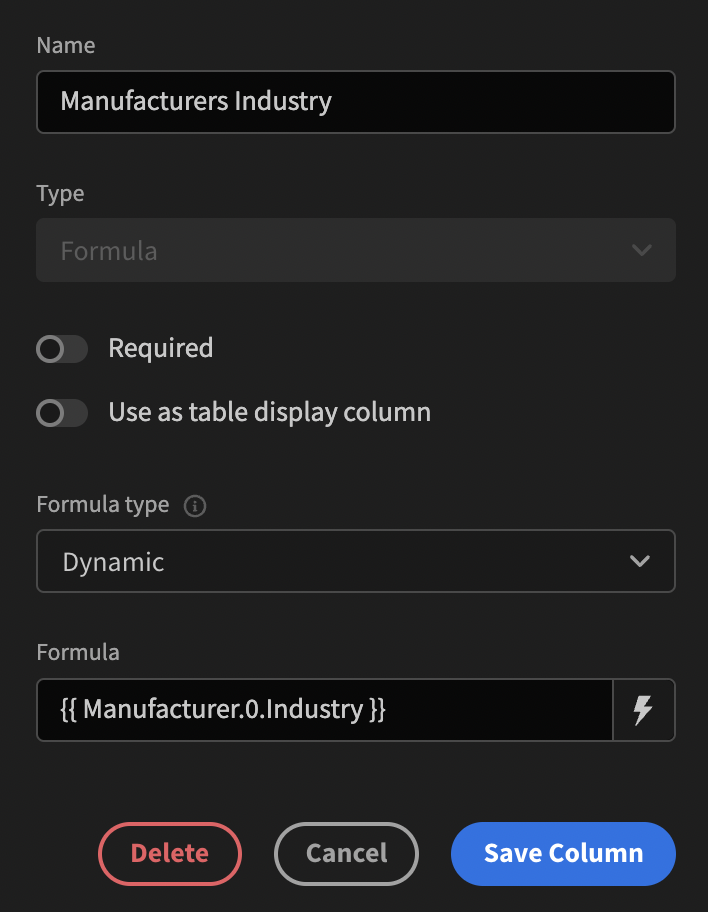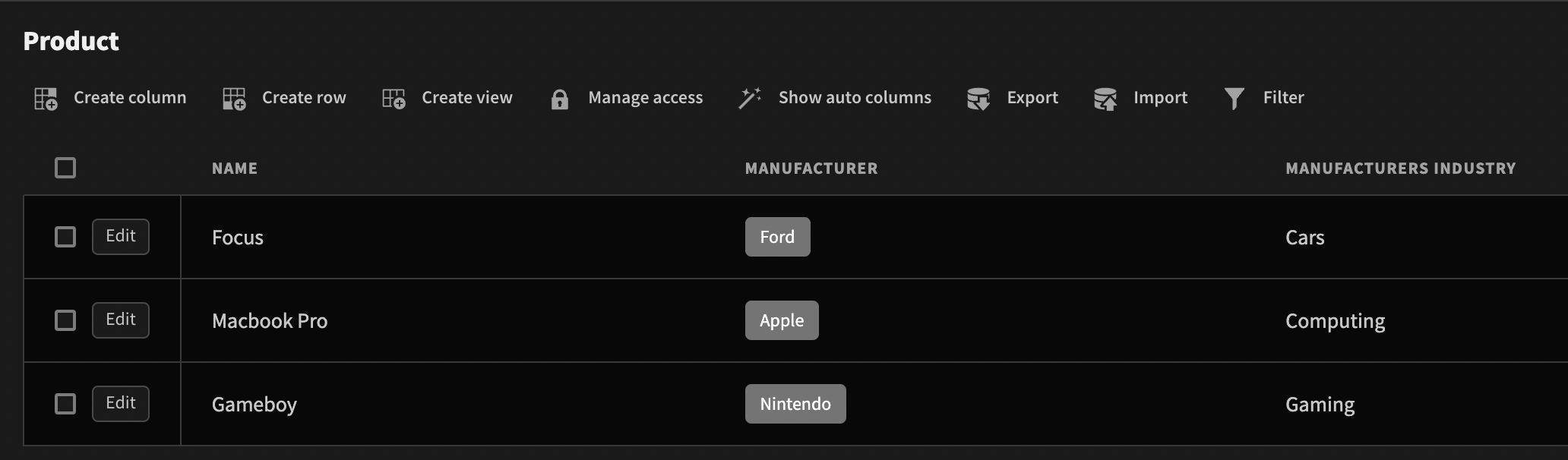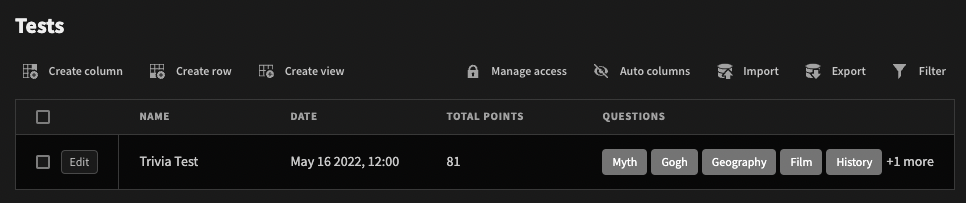# Formula

Generating a value from an expression

Formula columns allow you to generate values from an expression.
For example, you may wish to concatenate first and last names.

Just like with the other data types, start by creating a new column. Select Formula and you will be presented with a textbox. Use Handlebars to construct the expression you are interested in and hit the Save Column button.

If you want some assistance when writing your expression you can click the lightning bolt icon to the right of the formula field. This will open a modal where you can select the fields and handlebars expressions you want directly.## Static formulas

The default type of formula is Dynamic, which means the formula will be calculated every time data is read

Static formulas on the other hand are calculated and every time a row is created or updated. Additionally, the static formulas values are themselves saved into the Budibase DB which allows them to be used for filtering.

External databases only support dynamic formulas.

## Fields from relationships

A common use case for formulas is to access fields from a linked table.
For example, you may have one manufacturer for many products, and in your products table you wish to display the manufacturers industry.

To do this, create a formula column in the products table and then grab the Industry field from the first linked manufacturer:## Tutorial: Test scores using sum

Show the total score of a test by summing each of the question scores.

#### Steps

1. Create a 'Questions' table and a 'Tests' table.
2. Establish a One Test -> Many Questions relationship.
3. Add a Number type column named 'Points' to the 'Questions' table.1. In the 'Tests' table, create a new Formula column named 'Total'. Enter the binding:
``````{{ sum (pluck Questions 'Points') }}
``````

The first handle bar expression to get evaluated is the pluck function. This takes the array of related Questions and maps it to an array of their 'Points' values.
Then the sum function simply adds up each value from that array of points.

The equivalent in JavaScript is:

``````return \$("Questions").map(q => q.Points).reduce((a, b) => a + b)
``````📘

Number typing

If you want to numerically sort on a formula field or use numeric operators in a filter for a static formula field, then you must use JavaScript bindings.
Handlebars always return a string type.